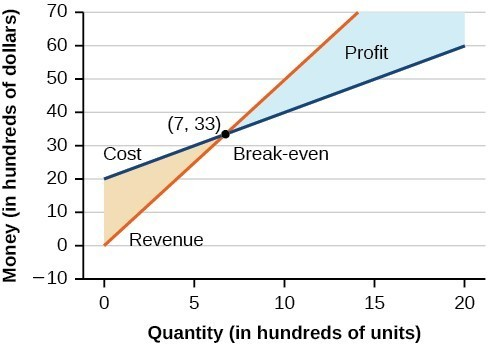## Cost and Revenue Problems

### Learning Outcomes

• Solve cost and revenue problems

A skateboard manufacturer introduces a new line of boards. The manufacturer tracks its costs, which is the amount it spends to produce the boards, and its revenue, which is the amount it earns through sales of its boards. How can the company determine if it is making a profit with its new line? How many skateboards must be produced and sold before a profit is possible?Using what we have learned about systems of equations, we can answer these questions. The skateboard manufacturer’s revenue equation is the equation used to calculate the amount of money that comes into the business. It can be represented as $y=xp$, where $x=$ quantity and $p=$ price. The revenue equation is shown in orange in the graph below.

The cost equation is the equation used to calculate the costs of doing business. It includes fixed costs, such as rent and salaries, and variable costs, such as utilities. The cost equation is shown in blue in the graph below. The $x$ -axis represents quantity in hundreds of units. The $y-axis$ represents both cost and revenue in hundreds of dollars. We won’t learn how to write a cost equation in this example, they will be given to you. If you take any business or economics courses, you will learn more about how to write a cost equation.The point at which the two lines intersect is called the break-even point, we learned that this is the solution to the system of linear equations that in this case comprise the cost and revenue equations.

Read the axes of the graph carefully, note that quantity is in hundreds, and money is in thousands. The solution to the graphed system is $(7, 33)$. This means that if $700$ units are produced, the cost to make them is $3,300$ and the revenue is also $3,300$. In other words, the company breaks even if they produce and sell $700$ units. They neither make money nor lose money.

The shaded region to the right of the break-even point represents quantities for which the company makes a profit. The shaded region to the left represents quantities for which the company suffers a loss.

### Example

A business wants to manufacture bike frames. Before they start production, they need to make sure they can make a profit with the materials and labor force they have. Their accountant has given them a cost equation of $y=0.85x+35,000$ and a revenue equation of $y=1.55x$:

1. Interpret $x$ and $y$ for the cost equation
2. Interpret $x$ and $y$ for the revenue equation

### Example

Given the same cost and revenue equations from the previous example, find the break-even point for the bike manufacturer.  Interpret the solution with words.

Cost: $y=0.85x+35,000$

Revenue: $y=1.55x$

### Try It

In our last video you will see an example of how to find the break even point for a small snow cone business.

We have seen that systems of linear equations and inequalities can help to define market behaviors that are very helpful to businesses.  The intersection of cost and revenue equations gives the break even point, and also helps define the region for which a company will make a profit.

## Contribute!

Did you have an idea for improving this content? We’d love your input.